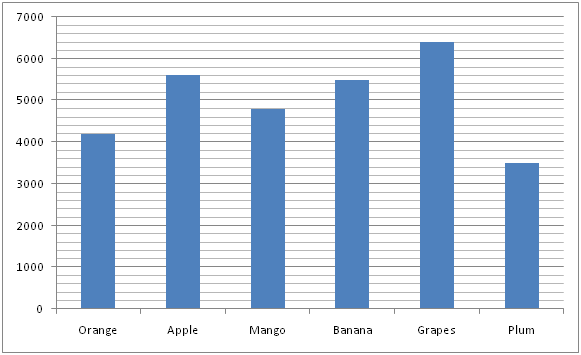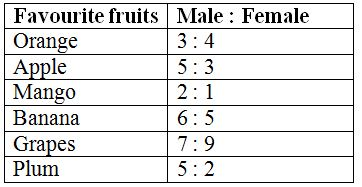# IBPS RRB PO Prelims Quantitative Aptitude Questions 2019 (Day-06)

Dear Aspirants, Our IBPS Guide team is providing new series of Quantitative Aptitude Questions for IBPS RRB PO Prelims 2019 so the aspirants can practice it on a daily basis. These questions are framed by our skilled experts after understanding your needs thoroughly. Aspirants can practice these new series questions daily to familiarize with the exact exam pattern and make your preparation effective.

Check here for IBPS RRB Prelims Mock Test 2019

[WpProQuiz 6617]

1) The speed of the man in still water is 12 km/hr and the rate of flow of water is 4 km/hr. He takes 9 hours to row to a place and come back. Find the distance between these two places?

a) 48 km

b) 72 km

c) 86 km

d) 64 km

e) None of these

2) A, B and C entered into a partnership with investment in the ratio of 6 : 7 : 9 respectively. After one year, B doubled his investment. At the end of two years, they earned a profit of Rs.59500. Find the share of B in the total profit?

a) Rs. 36800

b) Rs. 29400

c) Rs. 24500

d) Rs. 33200

e) None of these

3) A student got 48 % marks and failed by 12marks and another student got 62 % marks and get 16 more than the passing marks. Find the passing marks in the certain examination?

a) 126

b) 144

c) 132

d) 108

e) None of these

4) Divide Rs. 65000 into two parts such that the simple interest on the first part for 4 years at 12 % per annum is equal to the simple interest on the second part for 6 years at 5 % per annum. Find the two parts?

a) Rs. 33000 and Rs. 32000

b) Rs. 25000 and Rs. 40000

c) Rs. 20000 and Rs. 45000

d) Rs. 30000 and Rs. 35000

e) None of these

5) Two trains A and B having length of 300 m each running in opposite direction with a speed of 65 km/hr and 55 km/hr respectively can cross each other in how many seconds?

a) 24 sec

b) 16 sec

c) 26 sec

d) 20 sec

e) None of these

Directions (Q. 6 – 10): Study the following information carefully and answer the given questions?

The following bar graph shows the total number of peoples having 6 differentfavourite fruits in certain village.The table shows the ratio of male to that of female having those favourite fruits in the village.6) Find the ratio between the total number of male having a favourite fruit of Orange to that of total number of female having a favourite fruit of Plum?

a) 11 : 7

b) 9 : 5

c) 23 : 19

d) 37 : 28

e) None of these

7) Find the difference between the total number of male having a favourite fruits of Apple and Grapes together to that of total number of female having a favourite fruits of Mango and Banana together?

a) 2800

b) 3400

c) 3600

d) 2200

e) None of these

8) Find the average number of female having a favourite fruits of Apple, Mango, Banana and Grapes together?

a) 2450

b) 2600

c) 2250

d) 2700

e) None of these

9) Total number of peoples having a favourite fruits of Orange and Plum together is approximately what percentage of total number of peoples having a favourite fruits of Mango and Banana together?

a) 105 %

b) 120 %

c) 75 %

d) 60 %

e) 90 %

10) Total number of females having a favourite fruit of Plum is approximately what percentage less than the total number of males having a favourite fruit of Apple?

a) 86 %

b) 71 %

c) 57 %

d) 103 %

e) 120 %

Let the distance be x,

Speed of man in still water = 12 km/hr

Speed of stream = 4 km/hr

Speed of upstream = 12 – 4 = 8 km/hr

Speed of downstream = 12 + 4 = 16 km/hr

According to the question,

(x/16) + (x/8) = 9

(x + 2x)/16 = 9

x = 48 km

(Or)

Distance = Time * [(Speed of still water2 – Speed of stream2) / (2* Speed of still water)]

D = 9 * [(144 – 16) / (2 * 12)]

D = 9 * [128/24] = 48 km

The share of A, B and C

= > [6*2] : [7*1 + 14*1] : [9*2]

= >12 : 21 : 18

= >4 : 7 : 6

17’s = 59500

1’s = 3500

The share of B = Rs. 24500

Let the maximum mark be x,

Here passing mark is equal. So,

(48/100)*x + 12 = (62/100)*x – 16

(14/100)*x = 28

x = 28*(100/14) = 200

Passing mark = 200*(48/100) + 12 = 108

Let two parts be x and 65000 – x,

(x*4*12)/100 = [(65000 – x)*6*5]/100

48x = 1950000 – 30x

78x = 1950000

x = 1950000/78 = 25000

Two parts are Rs. 25000 and Rs. 40000

Time required to cross each other in,

=> (300+300) / [(65 + 55) * (5/18)]

= >[(600*18) / (120*5)] = 18 sec

Direction (6-10) :

The total number of male having a favourite fruit of Orange

= >4200*(3/7)

The total number of female having a favourite fruit of Plum

= >3500*(2/7)

Required ratio = [4200*(3/7)] : [3500*(2/7)] = 9 : 5

The total number of male having a favourite fruits of Apple and Grapes together

= >5600*(5/8) + 6400*(7/16)

= > 3500 + 2800 = 6300

The total number of female having a favourite fruits of Mango and Banana together

= >4800*(1/3) + 5500*(5/11)

= > 1600 + 2500 = 4100

Required difference = 6300 – 4100 = 2200

The average number of female having a favourite fruits of Apple, Mango, Banana and Grapes together

= >[5600*(3/8) + 4800*(1/3) + 5500*(5/11) + 6400*(9/16)] / 4

= > [2100 + 1600 + 2500 + 3600] / 4

= > 9800 / 4 = 2450

Total number of peoples having a favourite fruits of Orange and Plum together

= >4200 + 3500 = 7700

Total number of peoples having a favourite fruits of Mango and Banana together

= >4800 + 5500 = 10300

Required % = (7700 / 10300) * 100 = 74.75 % = 75 %

Total number of females having a favourite fruit of Plum

= >3500*(2/7) = 1000

Total number of males having a favourite fruit of Apple

= >5600*(5/8) = 3500

Required % = [(3500 – 1000) / 3500] * 100 = 71.42 % = 71 %